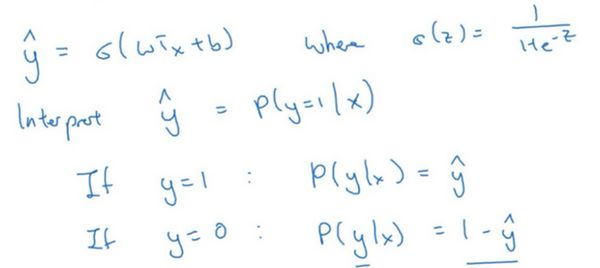# 深度学习笔记第一门课第二周：神经网络的编程基础（下）

### 2.9 逻辑回归中的梯度下降（Logistic Regression Gradient Descent）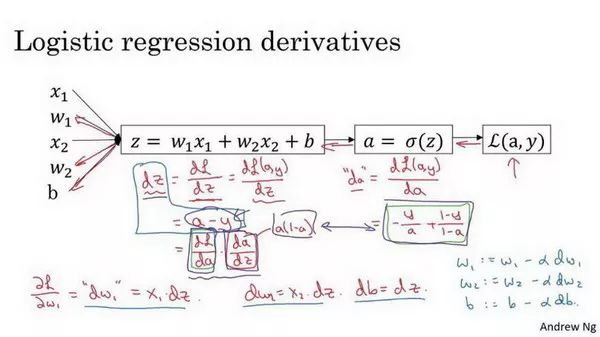### 2.10 m 个样本的梯度下降(Gradient Descent on m Examples)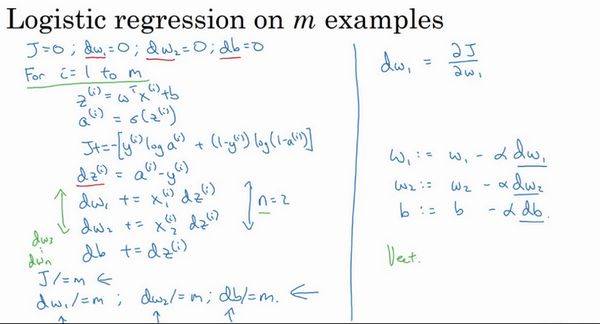J=0;dw1=0;dw2=0;db=0;
for i = 1 to m
z(i) = wx(i)+b;
a(i) = sigmoid(z(i));
J += -[y(i)log(a(i))+(1-y(i)）log(1-a(i));
dz(i) = a(i)-y(i);
dw1 += x1(i)dz(i);
dw2 += x2(i)dz(i);
db += dz(i);
J/= m;
dw1/= m;
dw2/= m;
db/= m;
w=w-alpha*dw
b=b-alpha*db


### 2.11 向量化(Vectorization)

z=0
for i in range(n_x):
z += w[i]*x[i]
z += b


z=np.dot(w,x)+b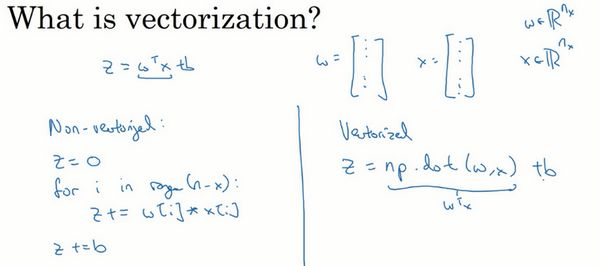import numpy as np #导入numpy库
a = np.array([1,2,3,4]) #创建一个数据a
print(a)
# [1 2 3 4]

import time #导入时间库
a = np.random.rand(1000000)
b = np.random.rand(1000000) #通过round随机得到两个一百万维度的数组
tic = time.time() #现在测量一下当前时间

#向量化的版本
c = np.dot(a,b)
toc = time.time()
print("Vectorized version:" + str(1000*(toc-tic)) +"ms") #打印一下向量化的版本的时间

#继续增加非向量化的版本
c = 0
tic = time.time()
for i in range(1000000):
c += a[i]*b[i]
toc = time.time()
print(c)
print("For loop:" + str(1000*(toc-tic)) + "ms")#打印for循环的版本的时间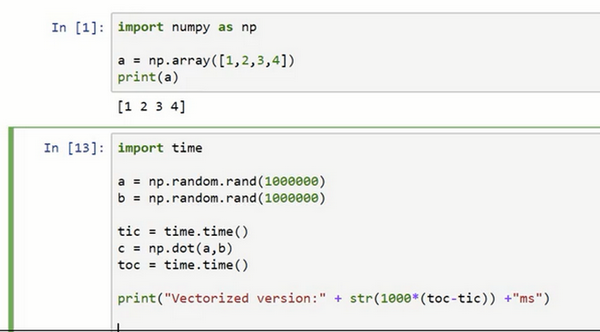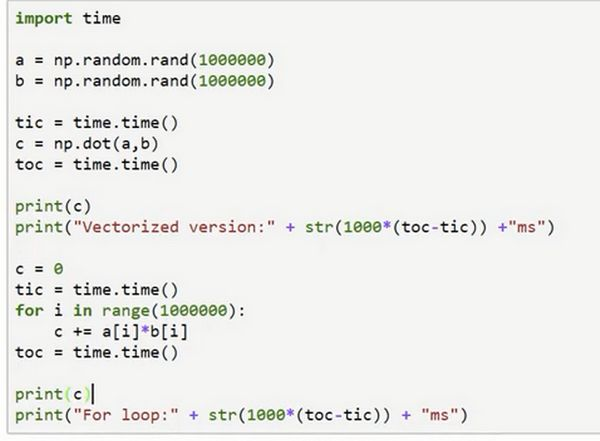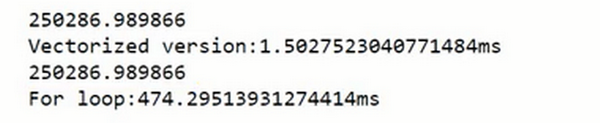### 2.12 向量化的更多例子（More Examples of Vectorization）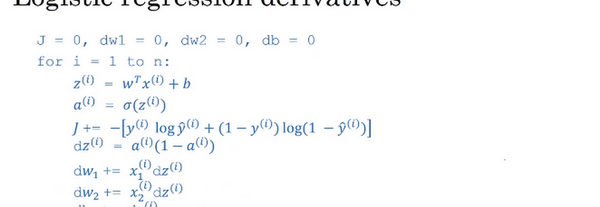### 2.13 向量化逻辑回归(Vectorizing Logistic Regression)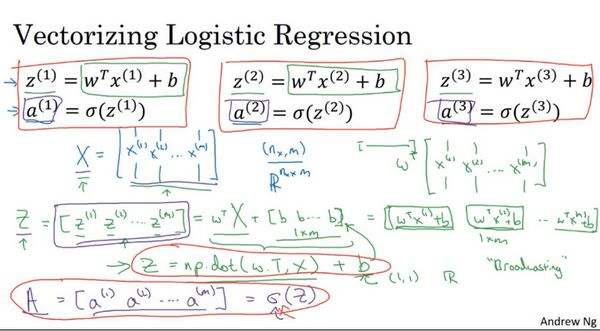这是第一个元素， 这是第二个元素，  这是第  个元素。

Z = np.dot(w.T,X) + b

### 2.14 向量化 logistic 回归的梯度输出（Vectorizing Logistic Regression's Gradient）

………….

………….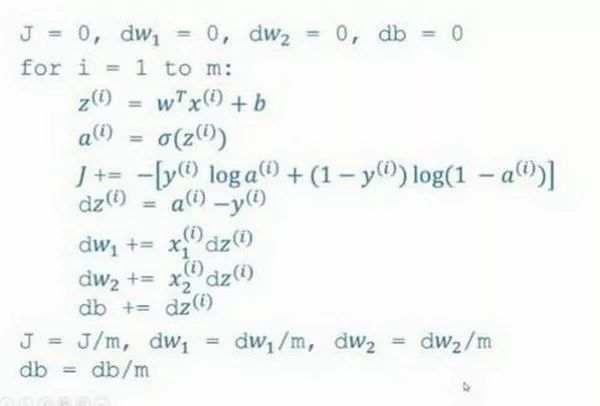### 2.15 Python 中的广播（Broadcasting in Python）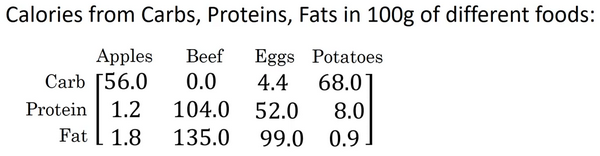jupyter notebook中输入如下代码，按shift+Enter运行，输出如下。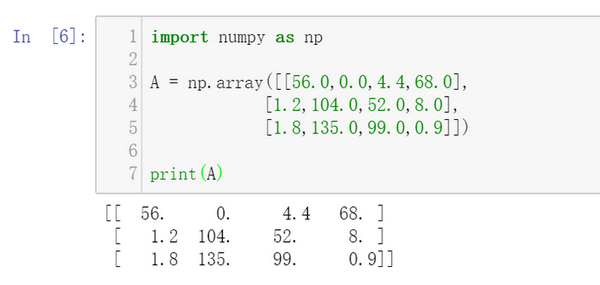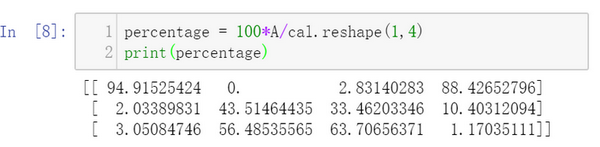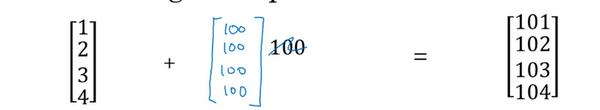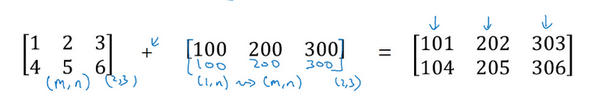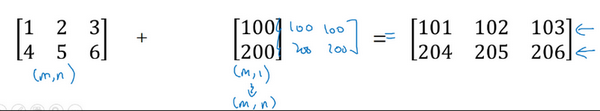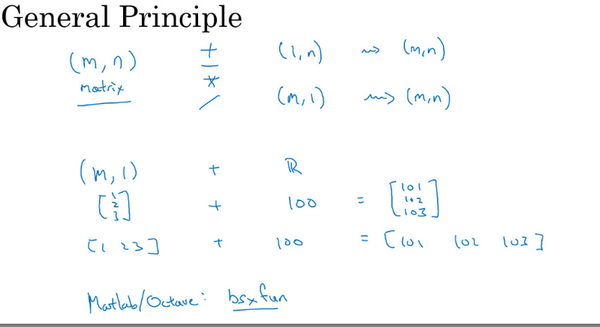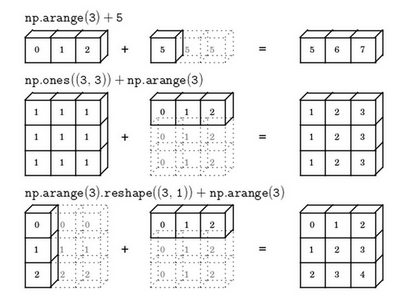### 2.16 关于 python _ numpy 向量的说明（A note on python or numpy vectors）参考视频：

Python的这些奇怪的影响之中，其实是有一个内在的逻辑关系的。但是如果对Python不熟悉的话，我就曾经见过的一些学生非常生硬、非常艰难地去寻找bug。所以我在这里想做的就是分享给你们一些技巧，这些技巧对我非常有用，它们能消除或者简化我的代码中所有看起来很奇怪的bug。同时我也希望通过这些技巧，你也能更容易地写没有bugPythonnumpy代码。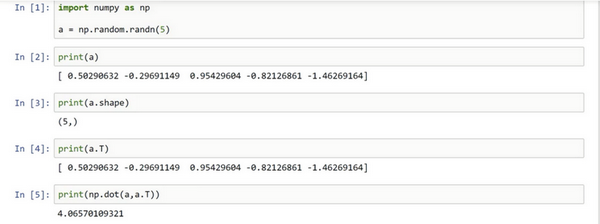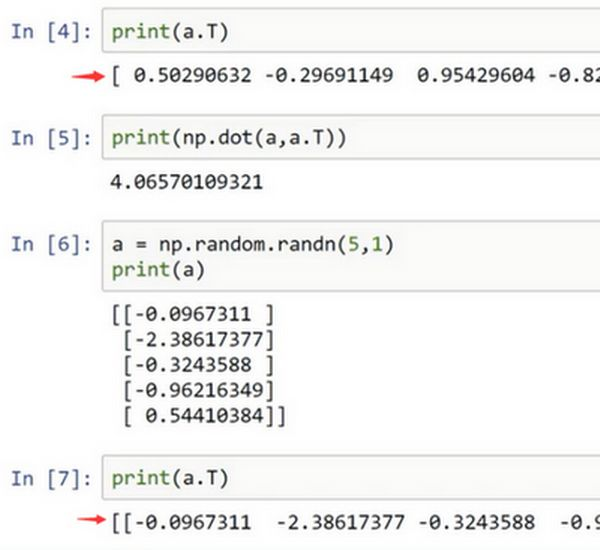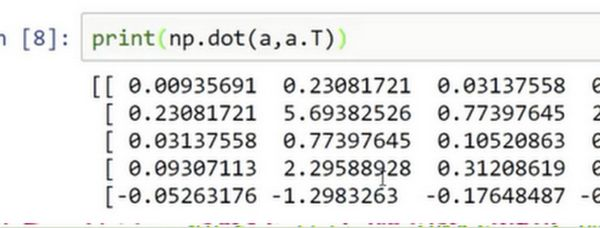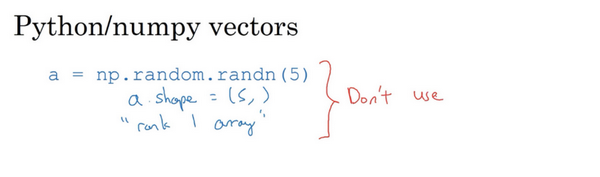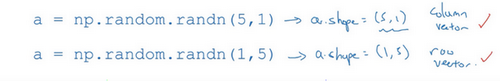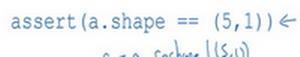### 2.17 Jupyter/iPython Notebooks 快速入门（Quick tour of Jupyter/iPython Notebooks）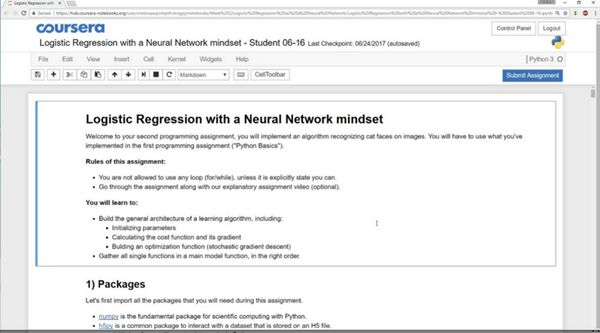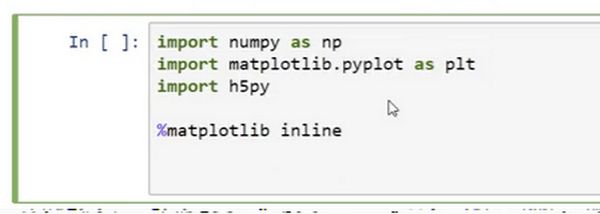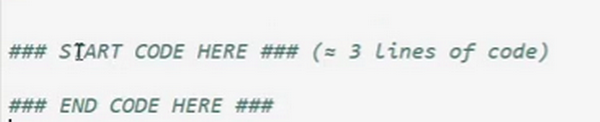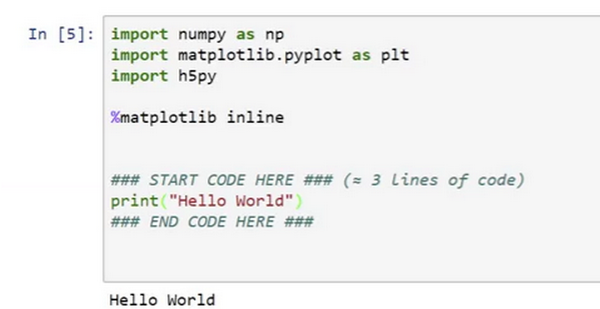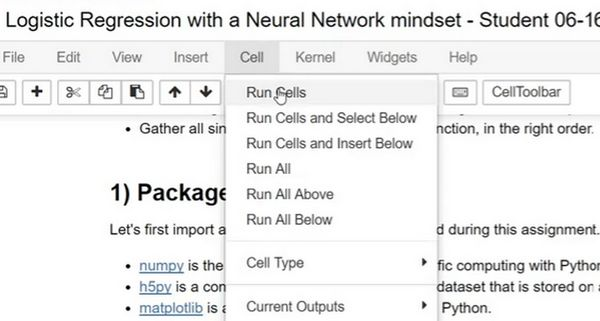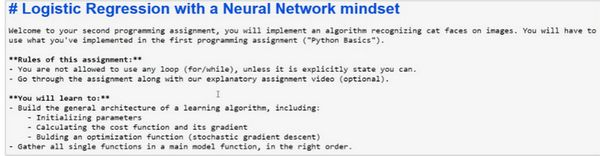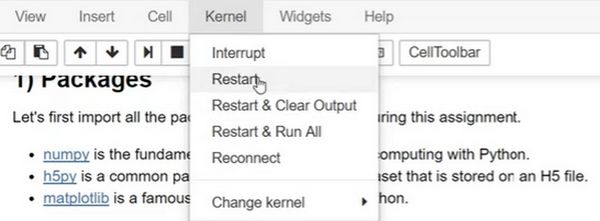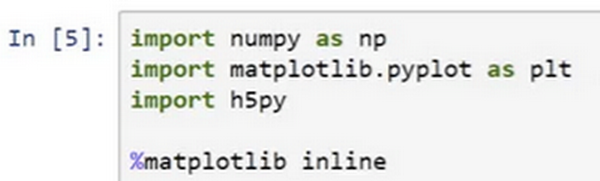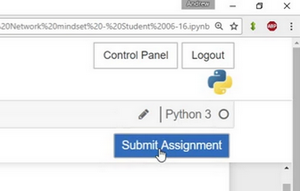### 2.18 （选修）logistic 损失函数的解释（Explanation of logistic regression cost function）